The patchwork package makes it very easy to create layouts in ggplot that have multiple panels.

The package is by Thomas Lin Pederson, and you can get it from his GitHub site:

https://github.com/thomasp85/patchwork

Installation

This is a little tricky, because the installation is not from CRAN. First, you need to install devtools, which is available from CRAN. Then load the devtools library and run the install_github command from the console:

# install.packages("devtools")
library(devtools)
install_github("thomasp85/patchwork")

I had a bit of trouble with this and ended up re-installing ggplot, but eventually it all worked.

Sample Data Sets

Here are some quick and dirty artificial data just to have something to plot:

library(ggplot2)
library(patchwork)

d1 <- runif(500)
d2 <- rep(c("Treatment","Control"),each=250)
d3 <- rbeta(500,shape1=100,shape2=3)
d4 <- d3 + rnorm(500,mean=0,sd=0.1)
plotData <- data.frame(d1,d2,d3,d4)
str(plotData)
## 'data.frame':    500 obs. of  4 variables:
##  \$ d1: num  0.628 0.506 0.298 0.169 0.227 ...
##  \$ d2: Factor w/ 2 levels "Control","Treatment": 2 2 2 2 2 2 2 2 2 2 ...
##  \$ d3: num  0.941 0.965 0.971 0.959 0.971 ...
##  \$ d4: num  0.934 0.988 0.732 1.119 0.913 ...

Create 4 Simple ggplot Graphs

p1 <- ggplot(data=plotData) + geom_point(aes(x=d3, y=d4))
p1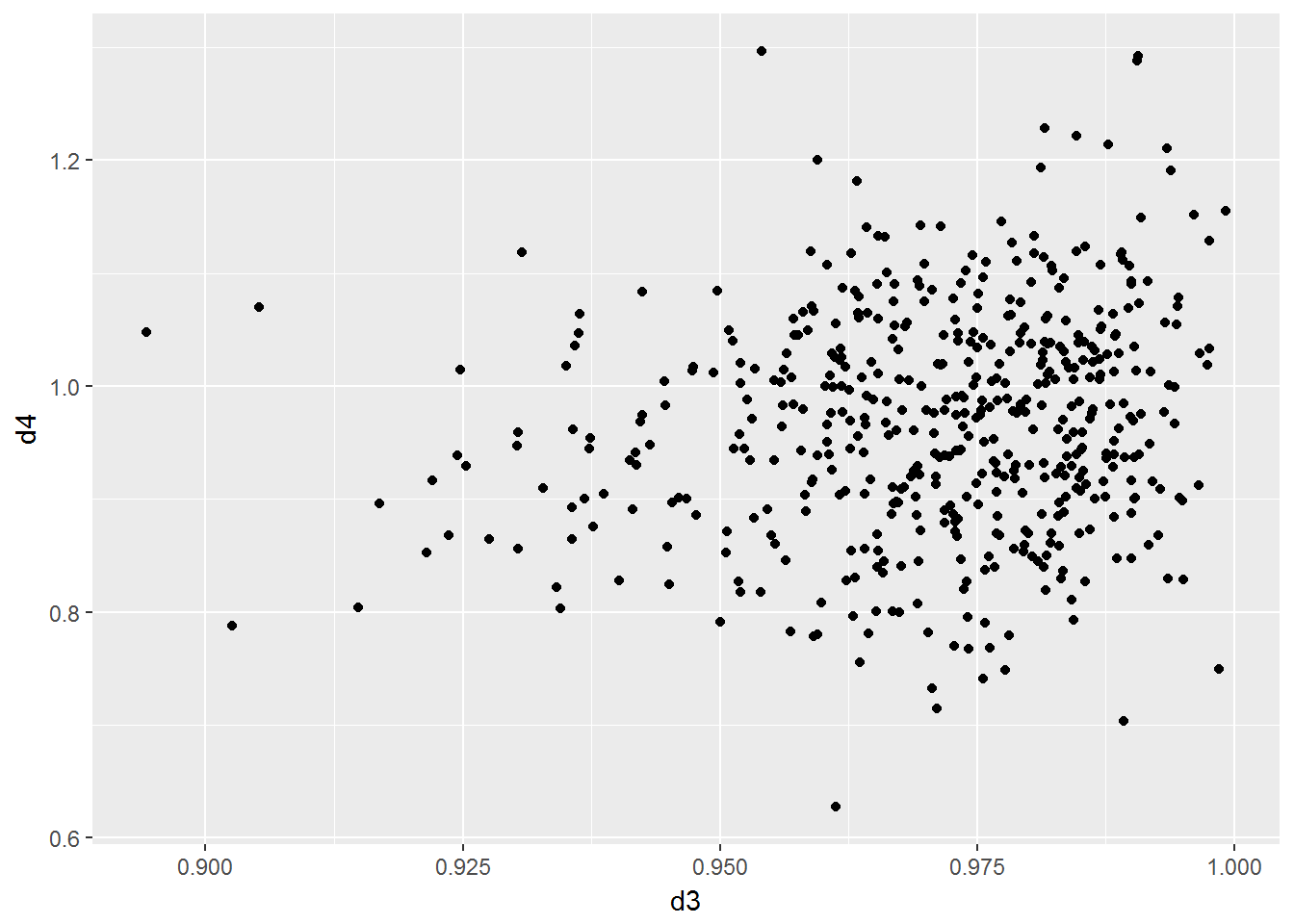p2 <- ggplot(data=plotData) + geom_boxplot(aes(x=d2,y=d1,fill=d2))+
theme(legend.position="none")
p2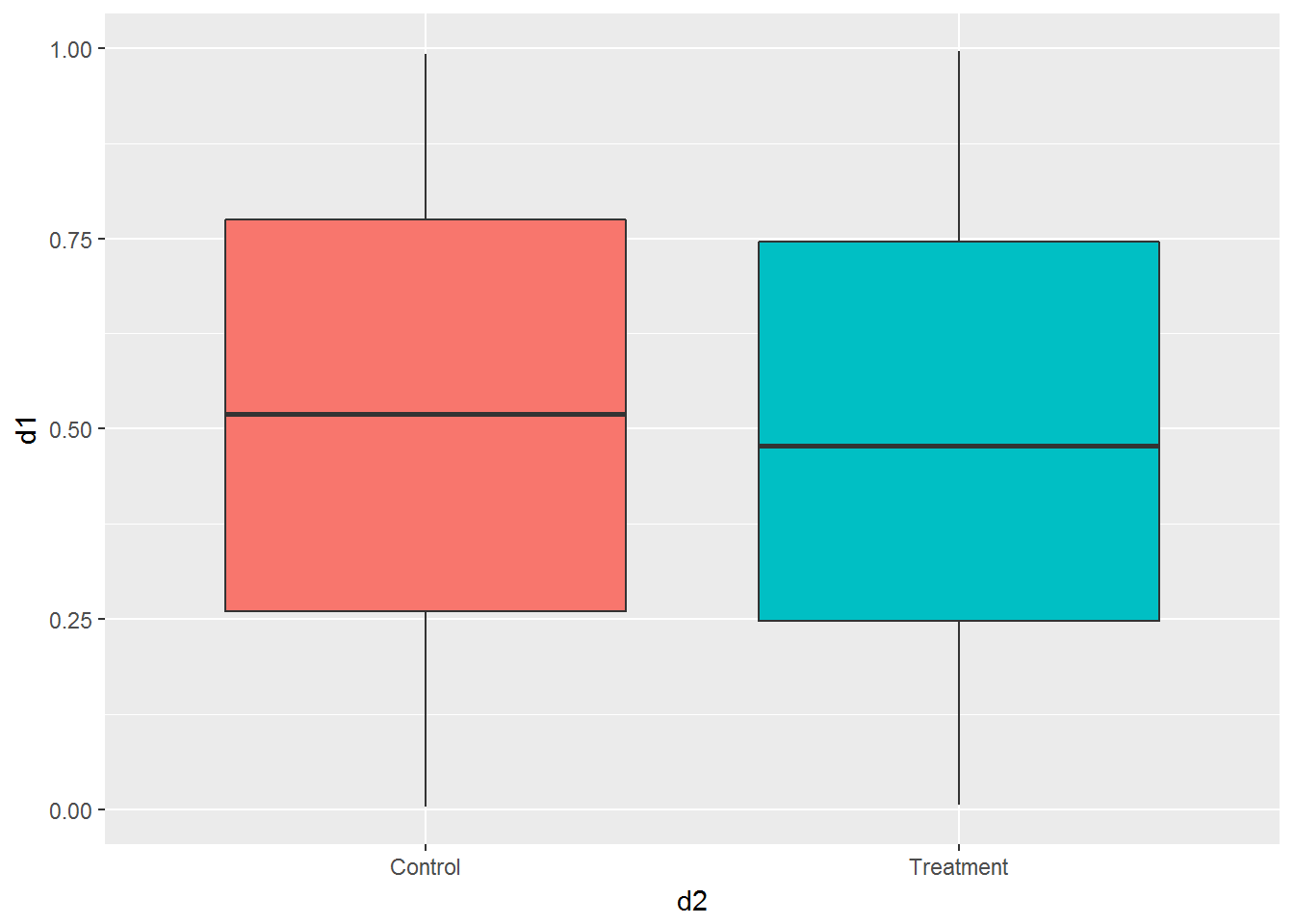p3 <- ggplot(data=plotData) +
geom_histogram(aes(x=d1, color=I("black"),fill=I("orchid")))
p3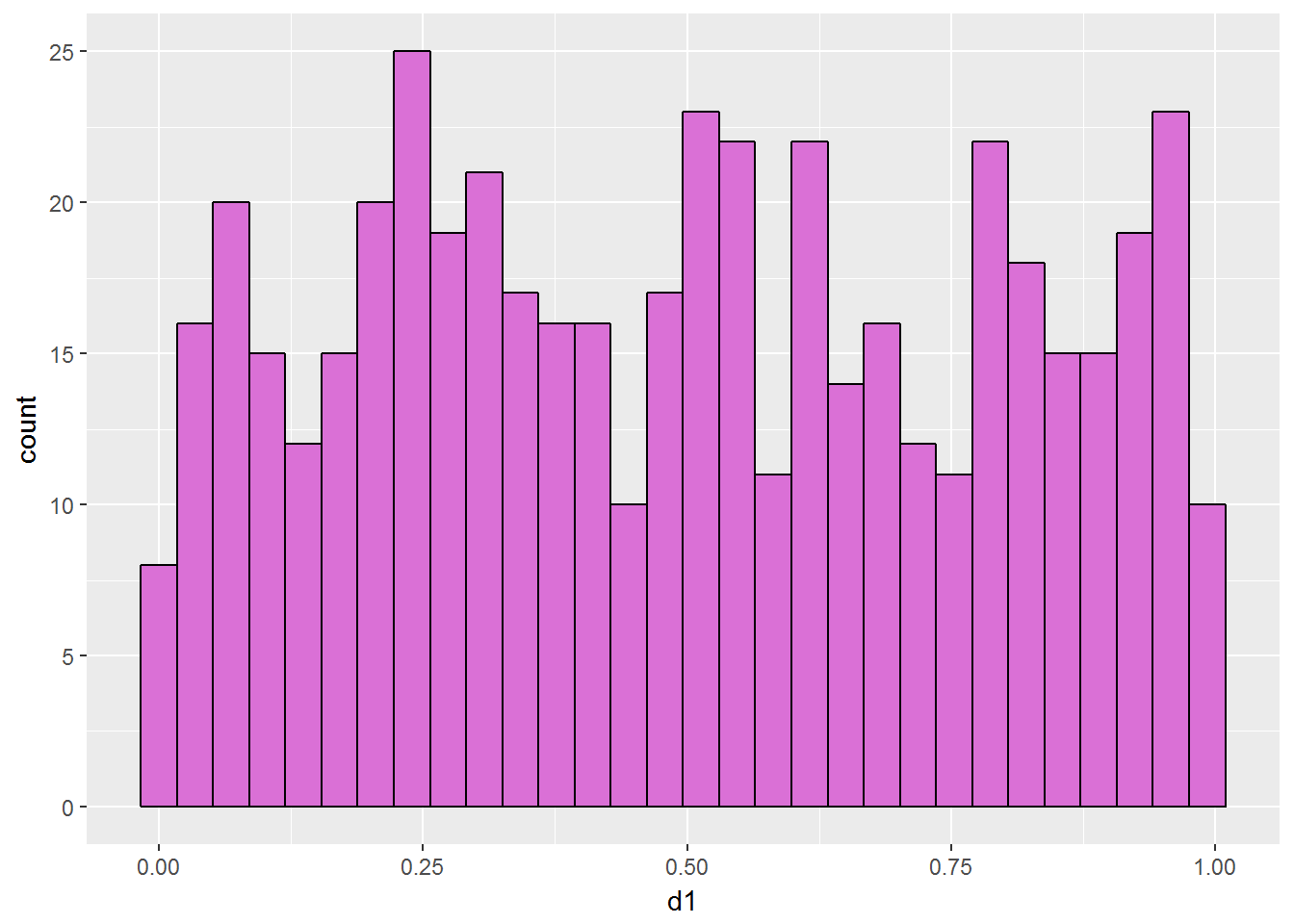p4 <- ggplot(data=plotData) +
geom_histogram(aes(x=d3, color=I("black"),fill=I("goldenrod")))
p4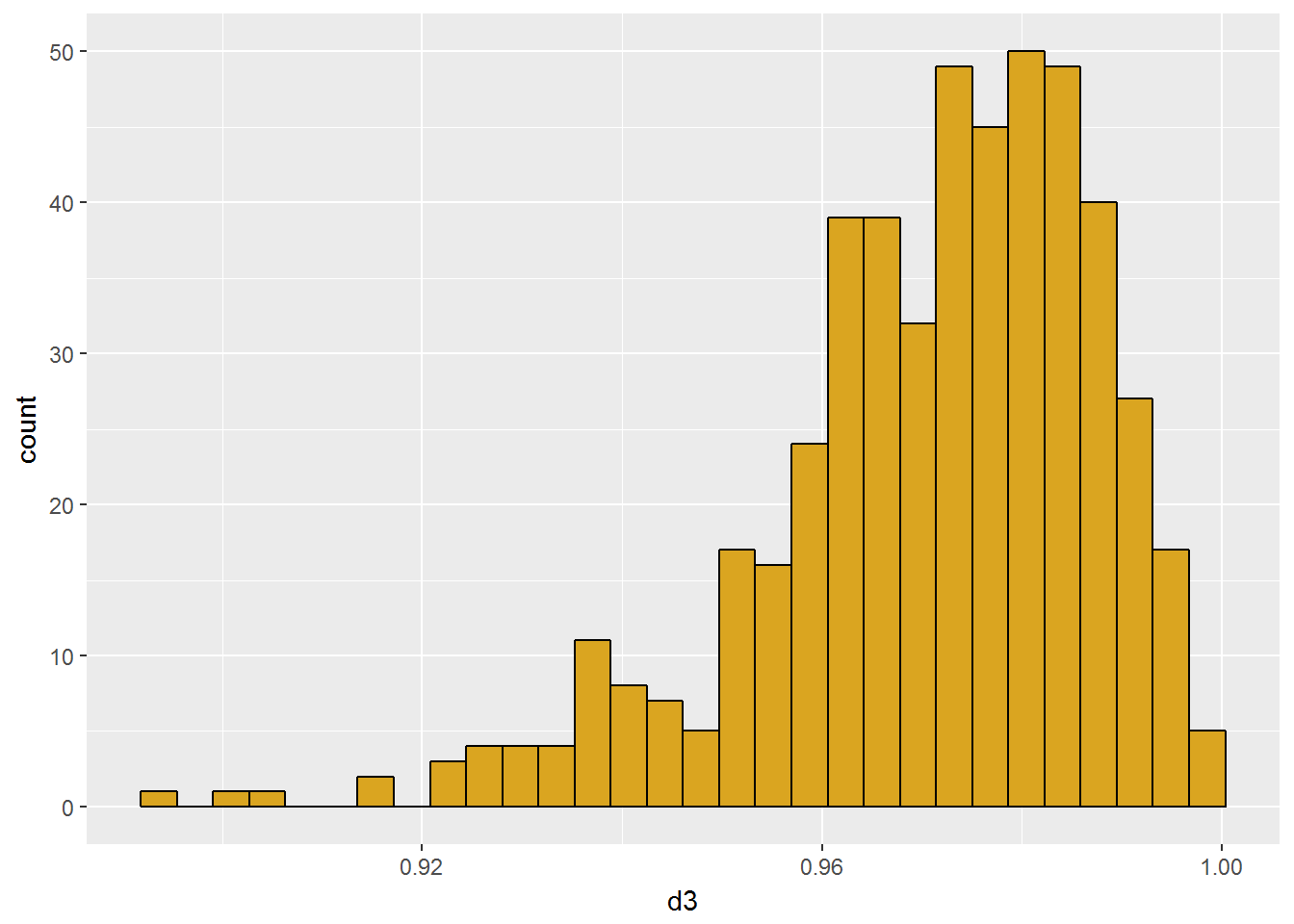Place Two Plots Horizontally

p1 + p2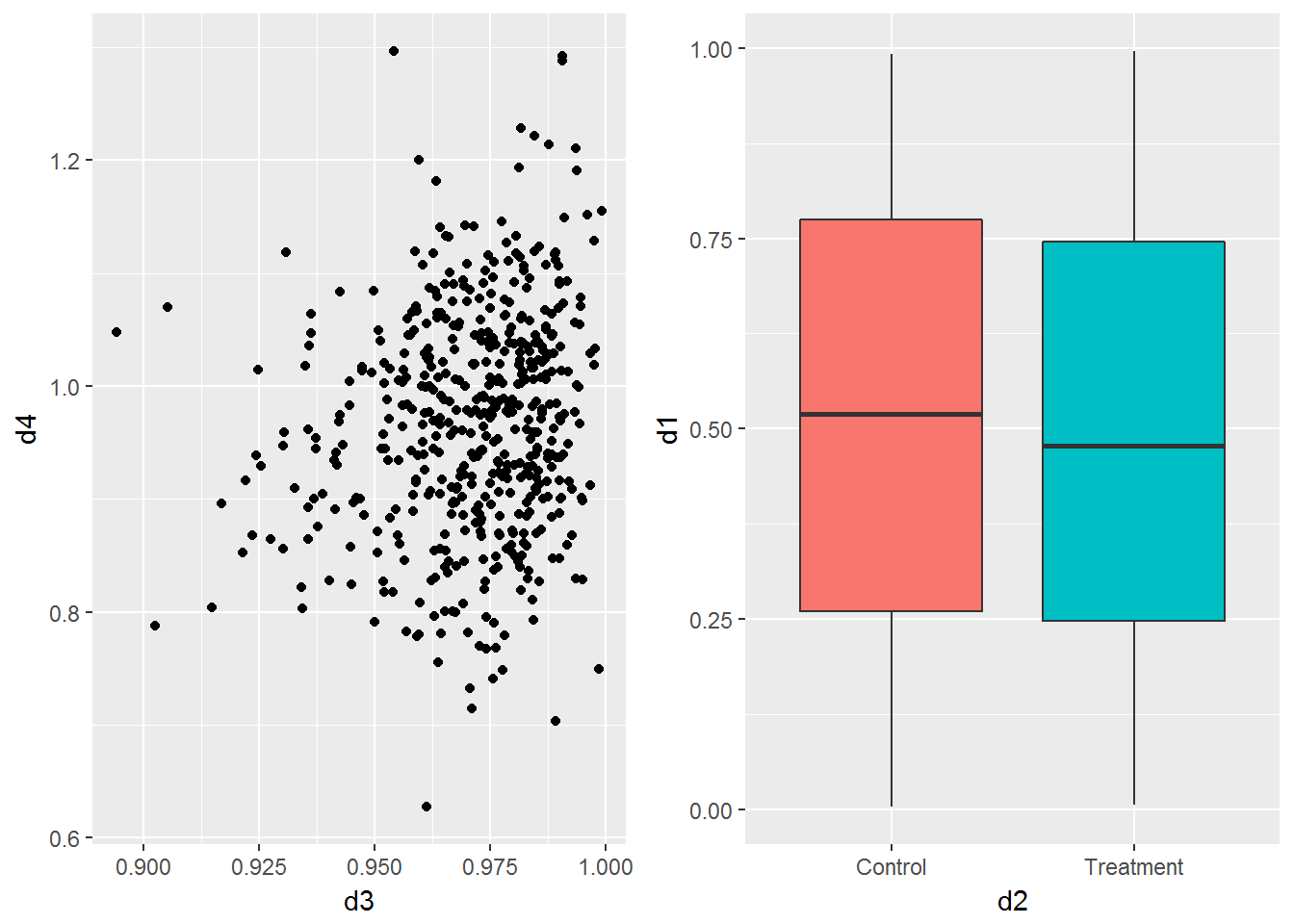Place Three Plots Vertically

p1 + p2 + p3 + plot_layout(ncol=1)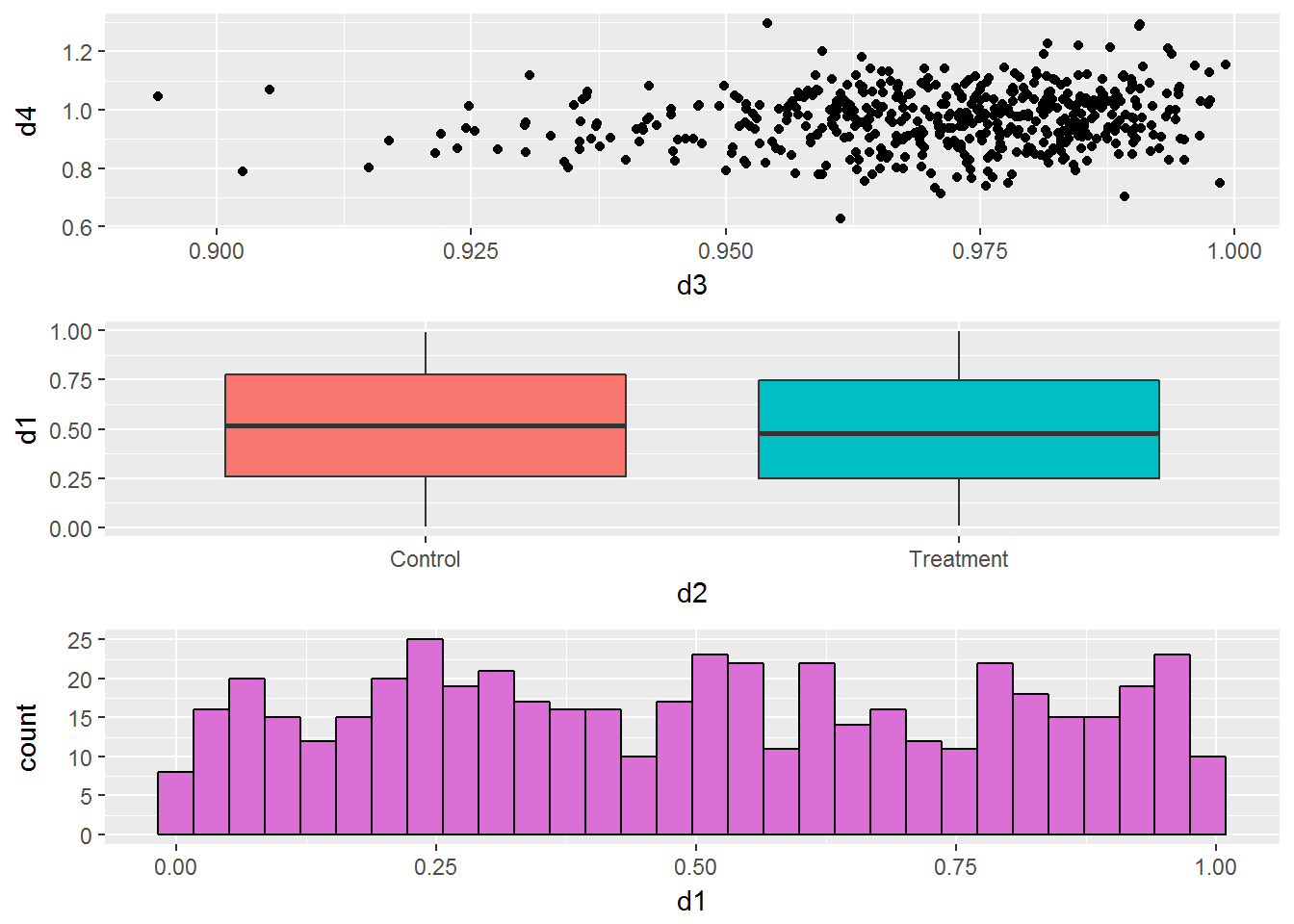Change Relative Area Of Each Plot

p1 + p2 + plot_layout(ncol=1,heights=c(2,1))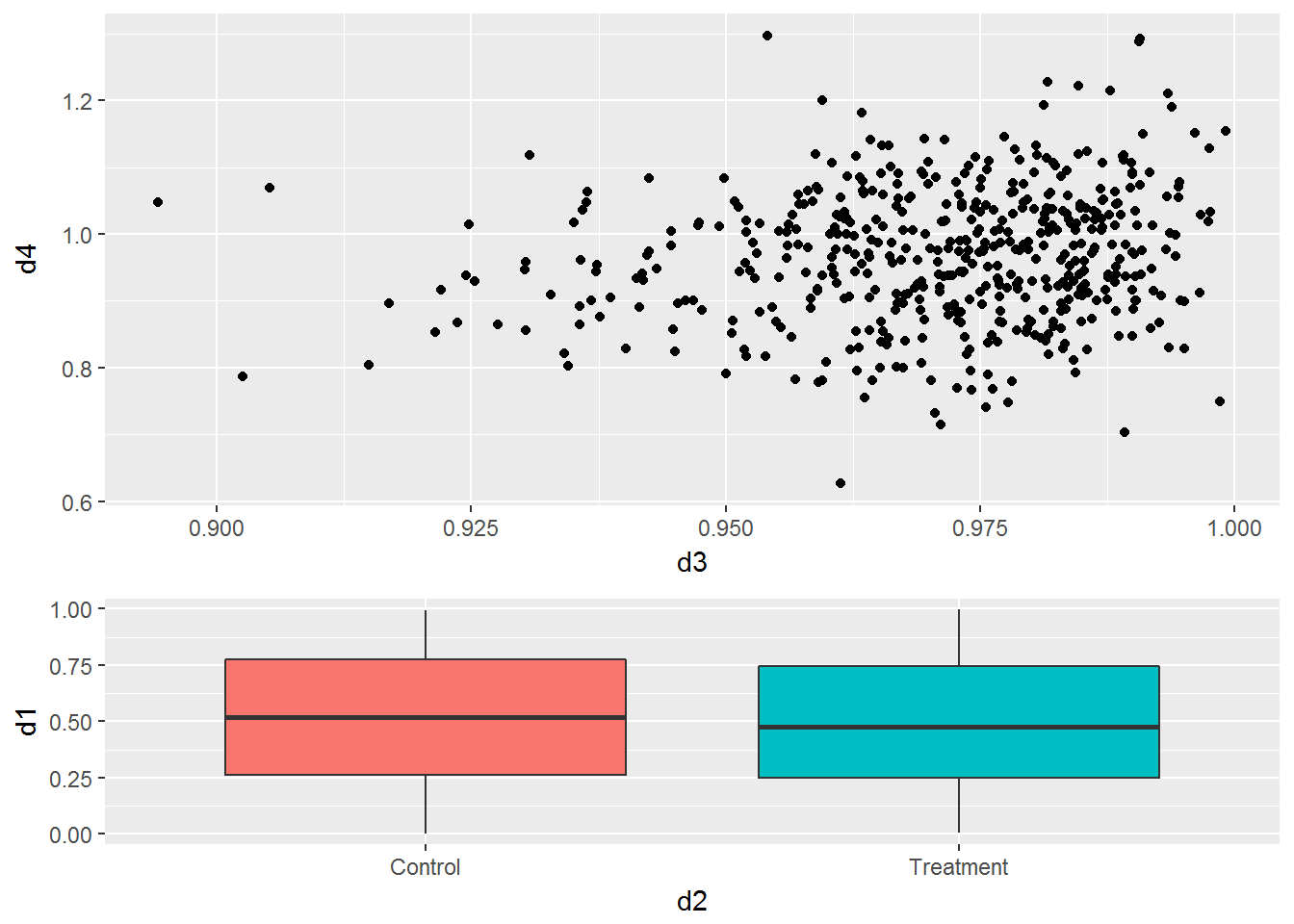p1 + p2 + plot_layout(ncol=2,widths=c(2,1))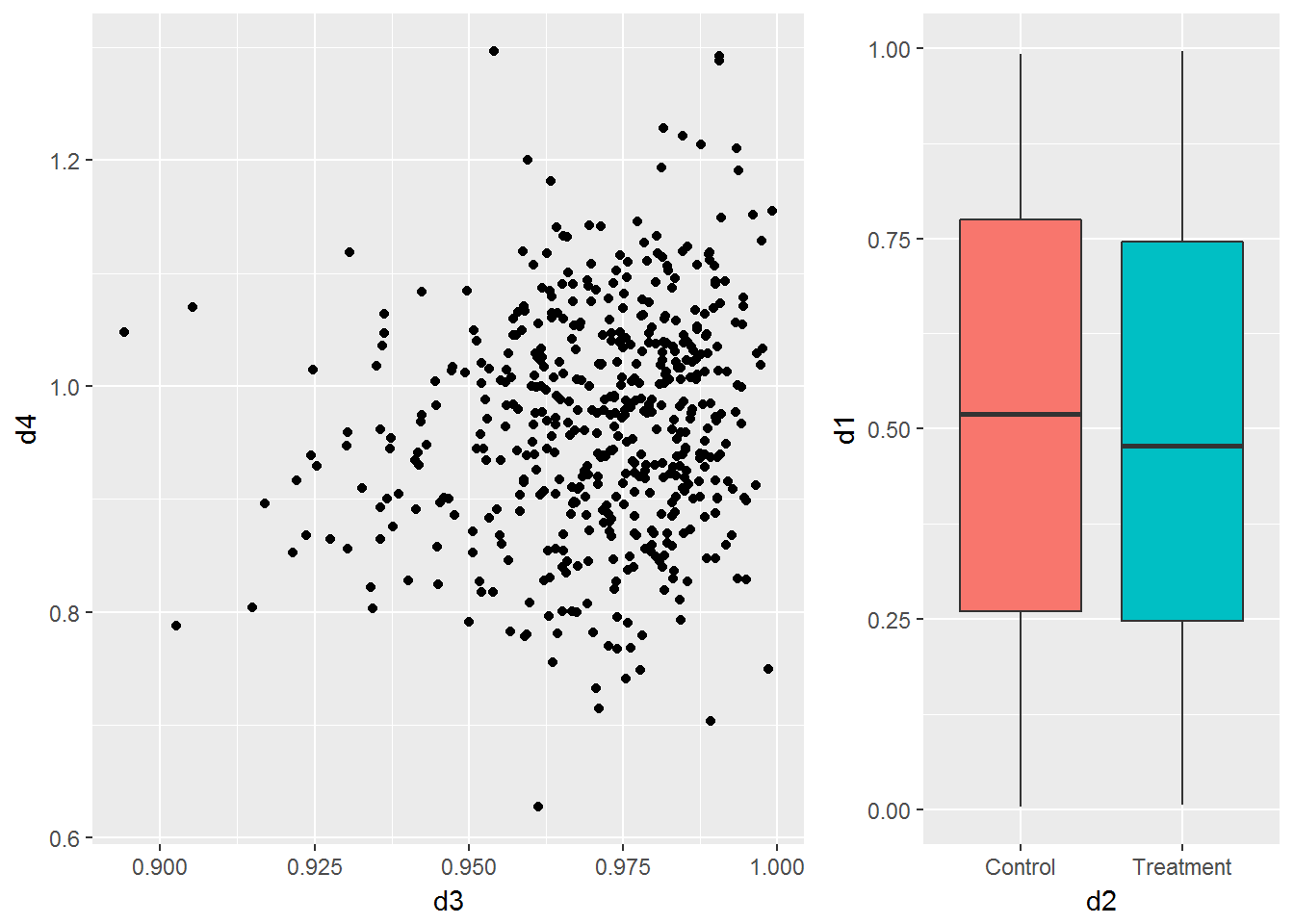# not working correctly!
p1 + plot_spacer() + p2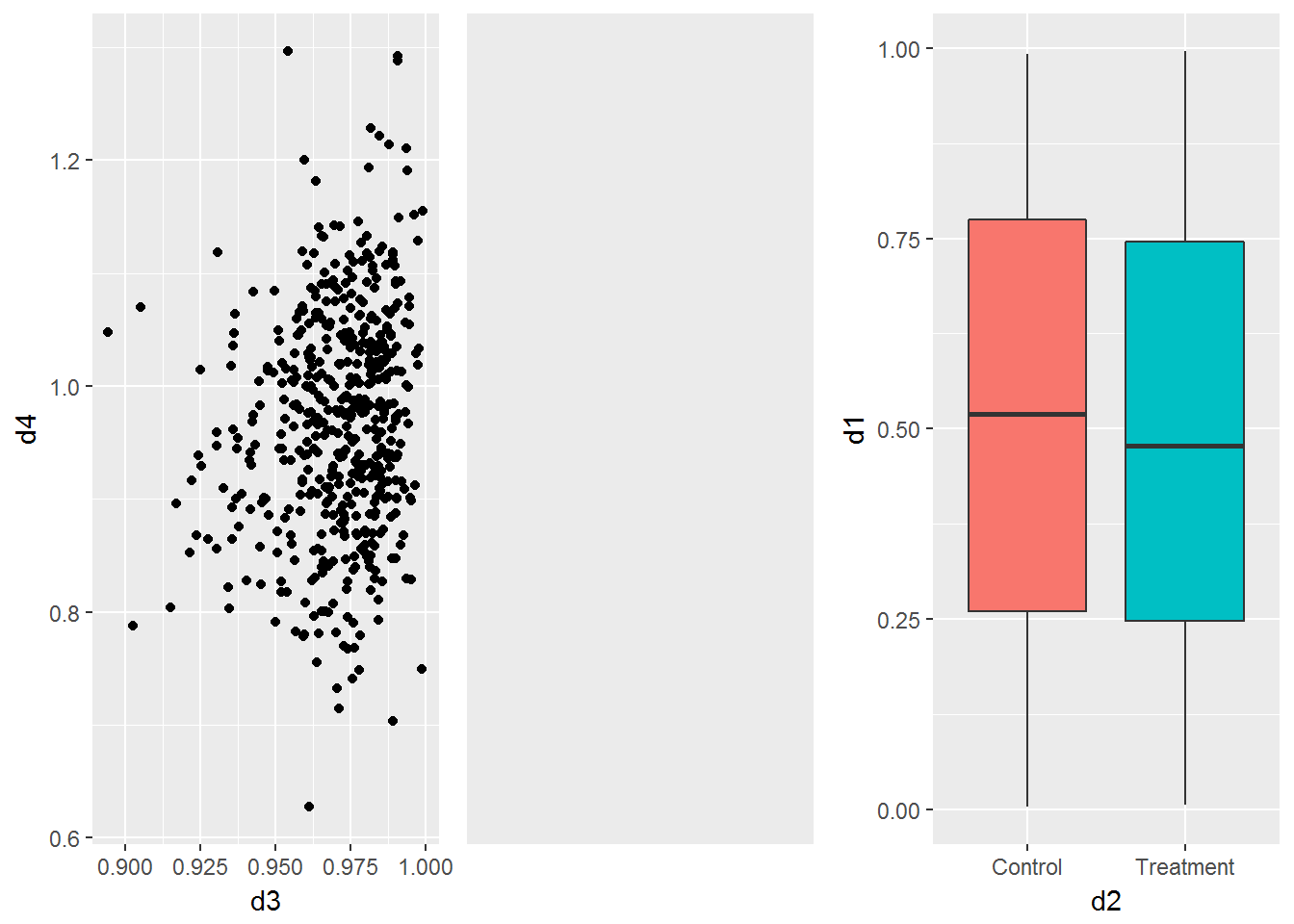Use Nested Layouts

p1 + {
p2 + {
p3 +
p4 +
plot_layout(ncol=1)
}
} +
plot_layout(ncol=1)